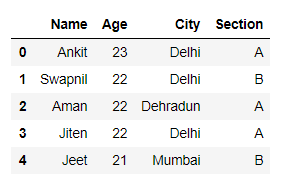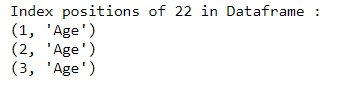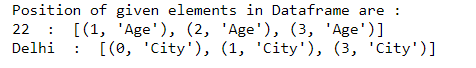GeeksforGeeks App
Open AppBrowser
Continue

# Find location of an element in Pandas dataframe in Python

In this article, we will see how to find the position of an element in the dataframe using a user-defined function. Let’s first Create a simple dataframe with a dictionary of lists, say column names are: ‘Name’, ‘Age’, ‘City’, and ‘Section’.

## Python3

 `# Import pandas library``import` `pandas as pd` `# List of tuples``students ``=` `[(``'Ankit'``, ``23``, ``'Delhi'``, ``'A'``),``            ``(``'Swapnil'``, ``22``, ``'Delhi'``, ``'B'``),``            ``(``'Aman'``, ``22``, ``'Dehradun'``, ``'A'``),``            ``(``'Jiten'``, ``22``, ``'Delhi'``, ``'A'``),``            ``(``'Jeet'``, ``21``, ``'Mumbai'``, ``'B'``)``            ``]` `# Creating Dataframe object``df ``=` `pd.DataFrame(students, columns ``=``[``'Name'``, ``'Age'``, ``'City'``, ``'Section'``])` `df`

Output:Example 1 : Find the location of an element in the dataframe.

## Python3

 `# Import pandas library``import` `pandas as pd` `# List of tuples``students ``=` `[(``'Ankit'``, ``23``, ``'Delhi'``, ``'A'``),``            ``(``'Swapnil'``, ``22``, ``'Delhi'``, ``'B'``),``            ``(``'Aman'``, ``22``, ``'Dehradun'``, ``'A'``),``            ``(``'Jiten'``, ``22``, ``'Delhi'``, ``'A'``),``            ``(``'Jeet'``, ``21``, ``'Mumbai'``, ``'B'``)``            ``]` `# Creating Dataframe object``df ``=` `pd.DataFrame(students, columns ``=``[``'Name'``, ``'Age'``, ``'City'``, ``'Section'``])` `# This function will return a list of``# positions where element exists``# in the dataframe.``def` `getIndexes(dfObj, value):``    ` `    ``# Empty list``    ``listOfPos ``=` `[]``    ` `    ``# isin() method will return a dataframe with``    ``# boolean values, True at the positions   ``    ``# where element exists``    ``result ``=` `dfObj.isin([value])``    ` `    ``# any() method will return``    ``# a boolean series``    ``seriesObj ``=` `result.``any``()` `    ``# Get list of column names where``    ``# element exists``    ``columnNames ``=` `list``(seriesObj[seriesObj ``=``=` `True``].index)``   ` `    ``# Iterate over the list of columns and``    ``# extract the row index where element exists``    ``for` `col ``in` `columnNames:``        ``rows ``=` `list``(result[col][result[col] ``=``=` `True``].index)` `        ``for` `row ``in` `rows:``            ``listOfPos.append((row, col))``            ` `    ``# This list contains a list tuples with``    ``# the index of element in the dataframe``    ``return` `listOfPos` `# Calling getIndexes() function to get``# the index positions of all occurrences``# of 22 in the dataframe``listOfPositions ``=` `getIndexes(df, ``22``)` `print``(``'Index positions of 22 in Dataframe : '``)` `# Printing the position``for` `i ``in` `range``(``len``(listOfPositions)):``    ``print``( listOfPositions[i])`

Output :Now let’s understand how the function getIndexes() works. The isin(), dataframe/series.any(), accepts values and returns a dataframe with boolean values. This boolean dataframe is of a similar size as the first original dataframe. The value is True at places where given element exists in the dataframe, otherwise False. Then find the names of columns that contain element 22. We can accomplish this by getting names of columns in the boolean dataframe which contains True. Now in the boolean dataframe we iterate over each of the selected columns and for each column, we find rows with True. Now, these combinations of column names and row indexes where True exists are the index positions of 22 in the dataframe. This is how getIndexes() founds the exact index positions of the given element & stores each position in the form of (row, column) tuple. Finally, it returns a list of tuples representing its index positions in the dataframe.
Example 2: Find location of multiple elements in the DataFrame.

## Python3

 `# Import pandas library``import` `pandas as pd` `# List of tuples``students ``=` `[(``'Ankit'``, ``23``, ``'Delhi'``, ``'A'``),``            ``(``'Swapnil'``, ``22``, ``'Delhi'``, ``'B'``),``            ``(``'Aman'``, ``22``, ``'Dehradun'``, ``'A'``),``            ``(``'Jiten'``, ``22``, ``'Delhi'``, ``'A'``),``            ``(``'Jeet'``, ``21``, ``'Mumbai'``, ``'B'``)``            ``]` `# Creating Dataframe object``df ``=` `pd.DataFrame(students, columns ``=``[``'Name'``, ``'Age'``, ``'City'``, ``'Section'``])` `# This function will return a``# list of positions where``# element exists in dataframe``def` `getIndexes(dfObj, value):``    ` `    ``# Empty list``    ``listOfPos ``=` `[]``    `  `    ``# isin() method will return a dataframe with``    ``# boolean values, True at the positions   ``    ``# where element exists``    ``result ``=` `dfObj.isin([value])``    ` `    ``# any() method will return``    ``# a boolean series``    ``seriesObj ``=` `result.``any``()` `    ``# Get list of columns where element exists``    ``columnNames ``=` `list``(seriesObj[seriesObj ``=``=` `True``].index)``   ` `    ``# Iterate over the list of columns and``    ``# extract the row index where element exists``    ``for` `col ``in` `columnNames:``        ``rows ``=` `list``(result[col][result[col] ``=``=` `True``].index)` `        ``for` `row ``in` `rows:``            ``listOfPos.append((row, col))``            ` `    ``# This list contains a list tuples with``    ``# the index of element in the dataframe``    ``return` `listOfPos` `# Create a list which contains all the elements``# whose index position you need to find``listOfElems ``=` `[``22``, ``'Delhi'``]` `# Using dictionary comprehension to find``# index positions of multiple elements``# in dataframe``dictOfPos ``=` `{elem: getIndexes(df, elem) ``for` `elem ``in` `listOfElems}` `print``(``'Position of given elements in Dataframe are : '``)` `# Looping through key, value pairs``# in the dictionary``for` `key, value ``in` `dictOfPos.items():``    ``print``(key, ``' : '``, value)`

Output :My Personal Notes arrow_drop_up